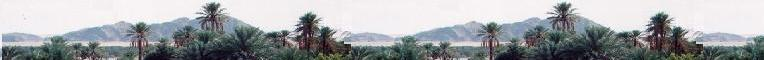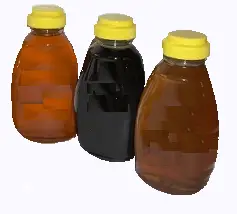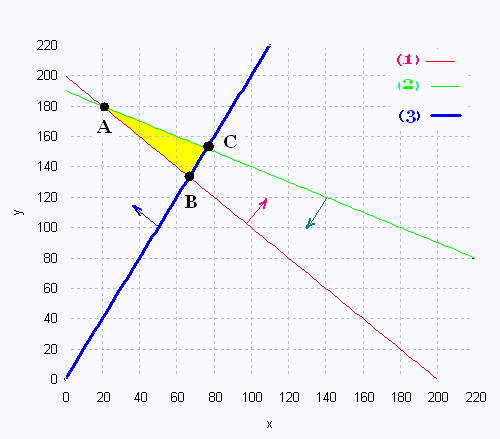# Mathematics 2: Linear optimization Examples

### HoneyAlbert sells three kinds of honey. Buckwheat, clover, and wildflower. He has no clover honey in stock. The Buckwheat honey is sold in 2-lbs bottles with 10 $, the wildflower honey is sold in 1-lb bottles with 12$. He needs at least 200 bottles to sell at most 380 lbs of honey, with at least 2 times 2-lbs bottles more than 1-lb bottles.
What is the maximum profit can Albert hope ?

Step 1: Identify the variables x, y
Step 2: formulate constraints and make the graph
Step 3: find vertices
Step 4: Write the equation to maximize profit
Step 5: Complete the table

step 1 :
x : number of 1-lb bottles
y : number of 2-lbs bottles
x ≥ 0
y ≥ 0
(1) x + y ≥ 200
(2) x + 2y ≤ 380
(3) y ≥ 2x

Step 2:
We translate the five constraints in a Cartesian plane.

Using the above inequality, we identify the polygon of constraints that will contain all the areas of each constraints.

Isolate the variables y in the three equations (1), (2), and (3):

(1) y ≥ - x + 200
(2) y ≤ - x/2 + 190
(3) y ≥ 2x

Plot the graph:The vertices of the polygon of constraints determine the minimum or maximum value of the function to be optimized. Comparison method will be useful to find some vertices.

From the polygon of constraints, we consider the three vertices. We will work with the equalities:

Vertex A:
This is the point of intersection between the constraints (1) and (2).
- x + 200 = - x/2 + 190
x = 20 and y = 180
The coordinates of the vertex A are (20, 180)

Vertex B:
This is the point of intersection between the constraints (1) and (3).
- x + 200 = 2x
x = 200/3 = 66.67 y = 400/3 = 133.33
The coordinates of the vertex B are (66.67 , 133.33)

Vertex C:
This is the point of intersection between the constraints (2) and (3).
- x/2 + 190 = 2x
x = 76 , y = 152
The coordinates of the vertex C are (76 , 152)

Step 4:
We write the equation that will use the found vertices and thus answer the question of the problem. We want the maximum profit:

The function objective Z is

Z = 12 x + 10 y

Step 5:
In a table, we use the function to optimize Z and perform a calculation using each found vertex.

 Vertex Z ($) (20, 180) 2040 (66.67, 133.33) 2133.34 (76 , 152) 2432 Step 6: We find one maximum value to maximize the profit. It is 2432$.

Answer: To get the maximum benefit, Albert will use 76 1-lb bottles, and 152 2-lbs bottles.Web ScientificSentence

 chimie labs | scientific sentence | java | php | green cat | contact |